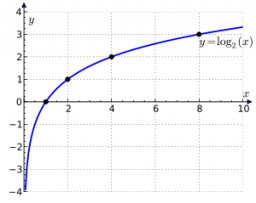# Simplify logarithm expr

Given that logxU + logxV =p and logxU - logxV =q

Prove that U=x^½(p+q)

Result

U = (Correct answer is: pow(x,(p+q)/2))### Step-by-step explanation:Did you find an error or inaccuracy? Feel free to write us. Thank you!